# A numerical method for solving an age structured virus model

Author: - 04 June 2019

Download Code

The aim of this tutorial is to give a numerical method for solving an age structured virus model.

## Introduction

The virus infection mathematical models are proposing and studying for a long time (see for example C.L. Althaus, R.J. DE Boer  and F. Brauer, C. Castillo-Chavez . In particular case of an HIV infection model (see for P. W. Nelson and al. ), the corresponding model is usually divided into three classes called uninfected cells, $T$, infected cells, $i$ and free virus particles, $V$.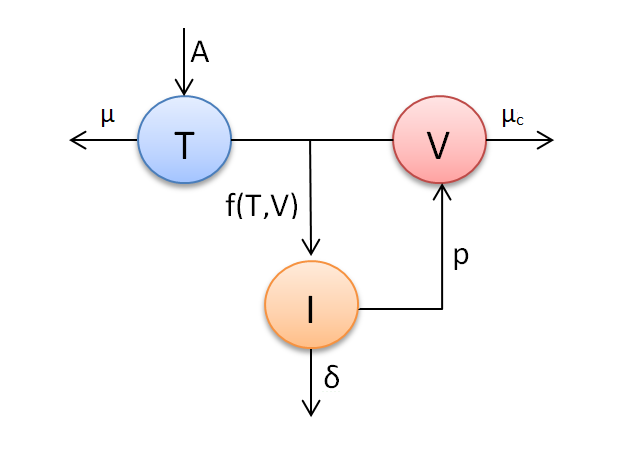schematic representation of HIV dynamic.

We refer the reader to  and  for more general class on nonlinear incidence rates that take into account the saturation phenomenon.

We consider an age-structured HIV infection model with a very general nonlinear infection function

with the boundary and initial conditions

We denote by

The number $R_0$ represents the expected number of secondary infections produced by a single infected cell during its lifetime,

The complete global stability analysis for the system (\ref{A}) has been studied in . The system (\ref{A}) admits at most two equilibrium, from Theorem 3.1 and Theorem 5.2 in , we obtain the following result:

• If $R_0 \leqslant 1$, the disease free equilibrium $E_0=(\frac{A}{\mu},0,0)$ is globally asymptotically stable.

• If $R_0>1$, the positive infection equilibrium $E^{\ast}=(T^\ast,i^\ast,V^\ast)$ is globally asymptotically stable.

## Numerical method

We consider the Beddington-Deangelis function $f$ defined by

In this case, the basic reproduction number ${\cal R}_0$ is given by

Where $\pi(a)=\exp^{-\int_{0}^{a} \delta (s) ds}$ and $N=\int_{0}^{\infty} p(a) \pi(a) da$.

The numerical method to solve this system of equation is based on the upwind method for solving hyperbolic partial differential equation, (see ) called also the FTBS method (Forward-Time-Backward-Space), has first-order accuracy in both space and time. The CFL condition is necessary for the stability of numerical solutions. The ODEs are solving by explicit Euler method.

we use a grid with points $(x_j,t_n)$ defined by

with age step $\Delta a$ and time step $\Delta t$. Denoting, respectively, by $T^n$, $i^n_j$ and $V^n$ the numerical approximation of $T(t_n)$, $i(t_n,a_j)$ and $V(t_n)$, moreover,

$\left( \frac{\partial i}{\partial t} \right)_n \simeq \frac{i^{n+1}_j-i^n_{j}}{\Delta t}, \qquad \left( \frac{\partial i}{\partial a} \right)_j \simeq \frac{i^n_j-u^n_{j-1}}{\Delta a}.$ we thus obtain the difference scheme of hyperbolic PDE

with $\lambda = \frac{\Delta t}{\Delta a}$. We fix the following values of parameters

with the initial conditions

The functions $p$ is given by

We change the values of $\tau_1$ in order to have $R_0 \leq 1$ or to have $R_0 > 1$. If we choose $\tau_1 = 3$ then $R_0 = 0.5417 < 1$,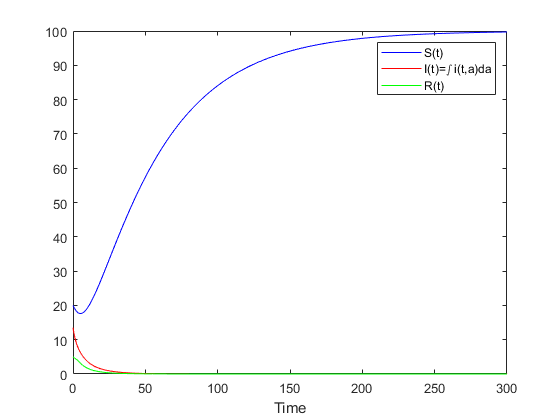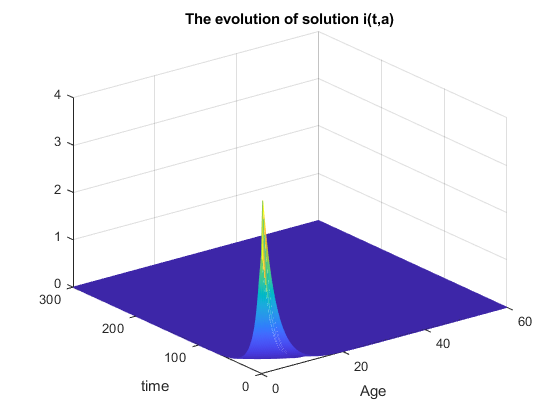And if we choose $\tau_1 = 0.5$ then $R_0 = 1.4216 > 1$,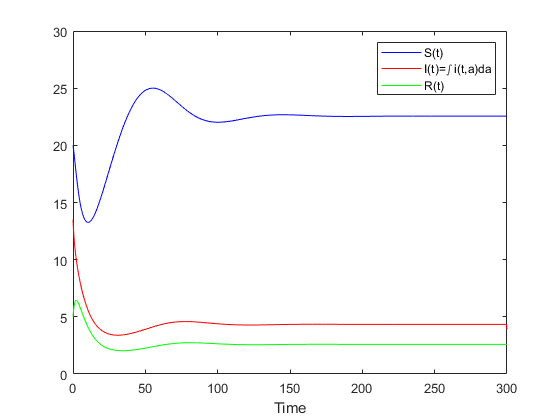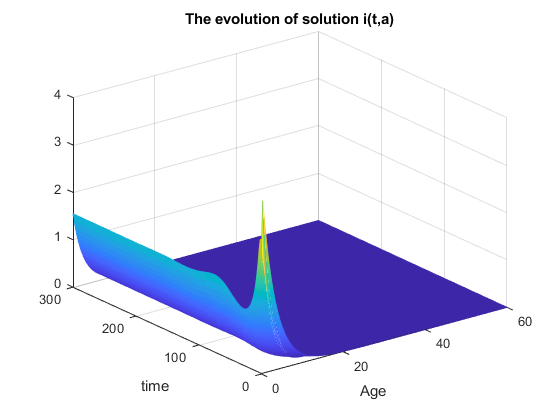clear all,close all
a1=0;
a2=60;
Tf=300;
I0=@(t)4*exp(-0.3*t);
T0=20;
V0=5;
A0=2; mu=0.02; muc=0.4;
beta=0.1;
alpha1=0.1;
alpha2=0.2;
delta=0.4;
fct=@(x,y) (beta*x*y)/(1+alpha1*x+alpha2*y);
N=199;M=1200;
h=(a2-a1)/N;
k=Tf/M;
a=a1+(1:N)*h;
ap=[0,a];
t=0+(0:M)*k;
lambda=k/h;
C=eye(N);
A=(1-lambda)*C+lambda*diag(ones(1,N-1),-1);


The reproduction number $R_0$

R0=tauxR(N,h,a,delta,beta,muc,mu,A0,alpha1)


R0 =

1.4216


T(1)=T0;
V(1)=V0;
U= I0(a(1:N))';  %% initial values
Up=[fct(T0,V0); U]; %% Initial value + Boundary condition
Uf=Up;

F=zeros(N,1);

UI=zeros(1,M);;

for j=1:M
Uold=U;
UI(j)=h*fct(T(j),V(j));
for o=1:N
UI(j)=UI(j)+h*Uold(o);
end
B=[lambda*fct(T(j),V(j)) ;zeros(N-1,1)];
F=- delta*Uold ;
U=A*Uold+B+k*F;
val=feval(@int,Uold,N-1,h,a);
T(j+1)=T(j)+k*(A0-mu*T(j)-fct(T(j),V(j)));
V(j+1)=V(j)+k*(val - muc *V(j));
Up=zeros(N+1,1);
Up=[fct(T(j+1),V(j+1)); U];
Uf=[Uf,Up];
end

 figure(1);
[X , Y] = meshgrid( ap ,t);
mesh (X , Y , Uf')
title('The evolution of solution i(t,a)')
xlabel('Age')
ylabel('time')
figure(2)
plot(t,T','b',t,[UI UI(N+2)],'r',t,V','g')
legend('S(t)','I(t)=\int i(t,a)da','R(t)')
xlabel('Time')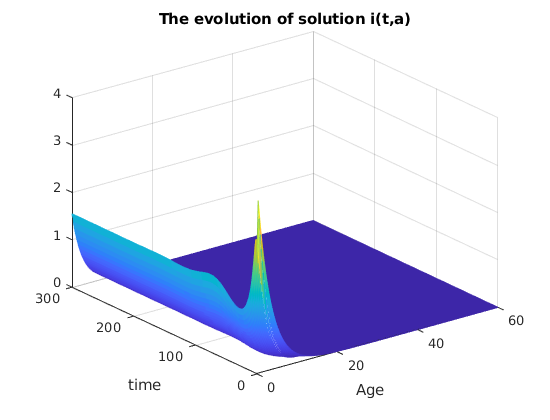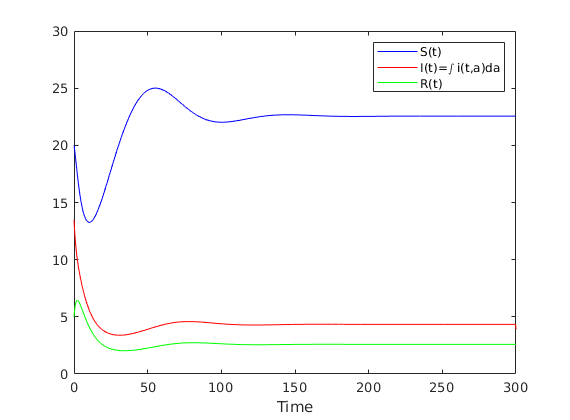R. J. De Boer C. L. Althaus. Dynamics of immune escape during hiv/siv infection, PloS Comput. Biol, 2008.

 F. Brauer and C. Castillo-Chavez. Mathematical Models in Population Biology and Epidemiology Springer, New York, 2000.

 L. Edsberg. Introduction to Computation and Modeling for Differential Equations, 2nd Edition. Wiley, 288 Pages, 2016.

 M. N. Frioui S. E. Miri, T. M. Touaoula. Unified lyapunov functional for an age-structured virus model with very general nonlinear infection response. J. Appl. Math. Comput, pages 1–27, 2017.

 T. Kuniya J. Wang, R. Zhang.. Mathematical analysis for an age-structured hiv infection model with saturation infection rate, Elect. J. Diff. Eq, pages 1–19, 2015.

 A. S. Perelson P. W. Nelson. Mathematical analysis of delay differential equation models of hiv-1 infection. Math. Biosc, 179, pages 73–94, 2002.